# Converting an Infinite Decimal Expansion to a Rational Number

Previous: Geometric Series Example

Next: Finding the Sum of an Infinite Series

## Question

Express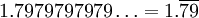as a ratio of integers.

## Complete Solution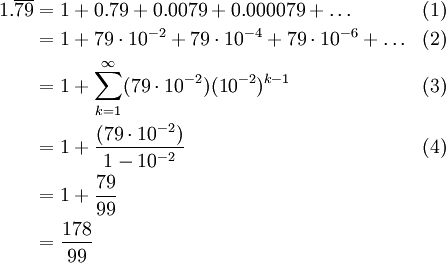## Explanation of Each Step

### Step (1)

Although not necessary, writing the repeating decimal expansion into a few terms of an infinite sum allows us to see more clearly what we need to do: relate each term to each other in some way to write the given number using sigma notation.

### Step (2)

Each term in the sum is equal to 79 times 10 to a power. Explicitly writing out what these powers are helps us look for a pattern in the individual terms of our sum.

### Step (3)

Suppose we allow our infinite series to start with the term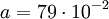. Then each term in the infinite series (after the second term) is related to its previous term by a factor of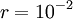. Using the form of the geometric series: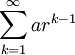and substituting our identified values for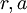into this formula yields Step (3).

### Step (4)

Here, we apply our formula for the sum of an infinite series,The rest of the problem is algebraic manipulation of fractions to find a simplified ratio of two integers.

## Possible Areas of Confusion

### Getting Started

Any problem of this type could be started in the same way: by writing out the first few terms of an infinite series. This way, it is easier to see a pattern in the terms of the infinite series.

Essentially, we solved the given problem by writing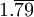as, which isolated the repeating digits, which can be written as a geometric series.

### Step (3)

Recall that our general form of the geometric series isIn Step (2), we can identify, and. We may substitute these values into the above general form to obtain Step (3).

Previous: Geometric Series Example

Next: Finding the Sum of an Infinite Series